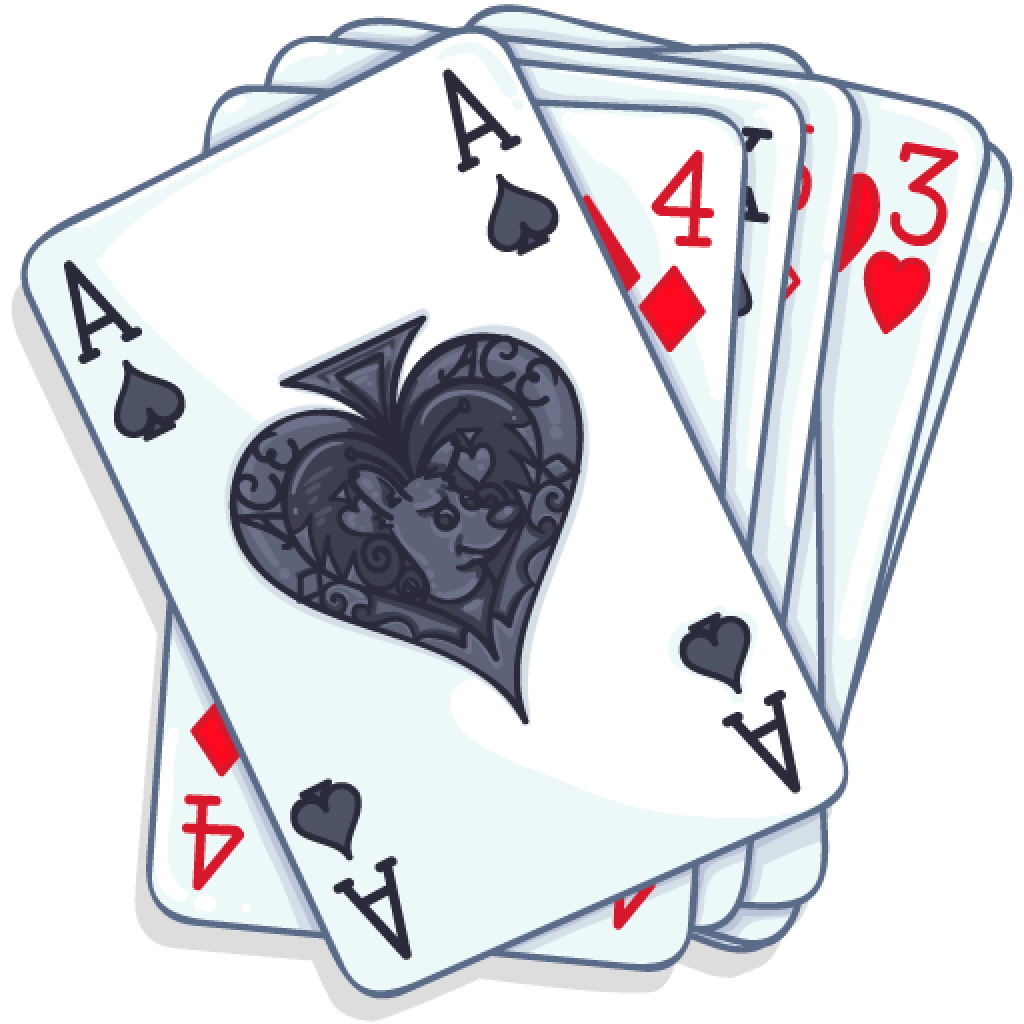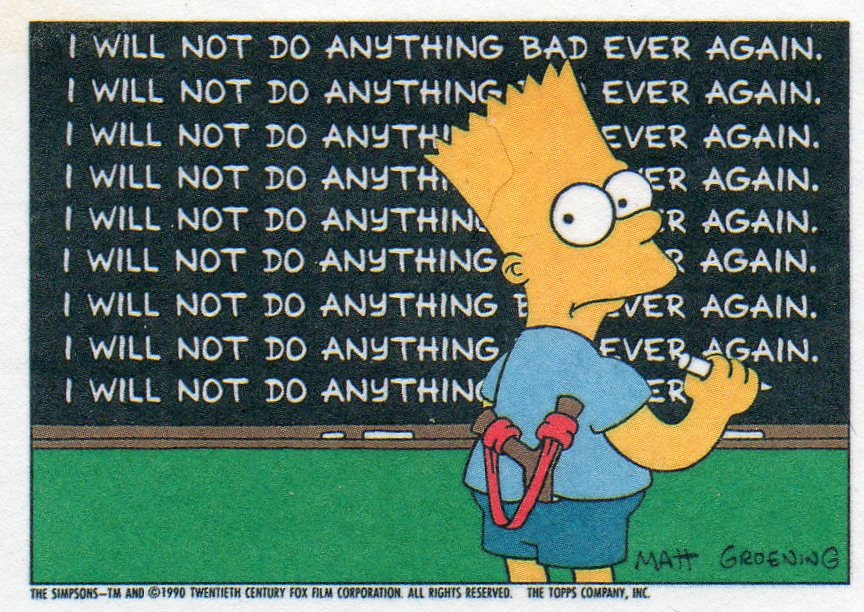# Lecture 16

### Machine Learning

Brooke Wenig + Guillaume Calmettes

## Monty Hall Problem## Monty Hall Problem

 What is really behind What the result will be Door 1 Door 2 Door 3 Staying at door 1 Switching to the door offered Car Goat Goat Wins car Wins goat Goat Car Goat Wins goat Wins car Goat Goat Car Wins goat Wins car Chance of getting the car: $1/3$ $2/3$

## What is Machine Learning?

Computer algorithms that learn patterns in your data.

Note:## Decision Making## Model this in Python?

        
if salary < 50k:
Decline offer
else if salary > 50k and commute > 1 hr
Decline offer



## Decision Tree

Really, it's an upside tree
(leaves on bottom)

Leaf:
Terminal node (no child)

Internal Node:
Splits the predictor space## Choosing a Restaurant

 Examples Attributes Target Wait Alt Bar Fri Hun Pat Price Rain Res Type Est $X_1$ T F F T Some F T French 0-10 T $X_2$ T F F T Full F F Thai 30-60 F $X_3$ F T F F Some F F Burger 0-10 T $X_4$ T F T T Full F F Thai 10-30 T $X_5$ T F T F Full F T French >60 F $X_6$ F T F T Some T T Italian 0-10 T $X_7$ F T F F None T F Burger 0-10 F $X_8$ F F F T Some T T Thai 0-10 T $X_9$ F T T F Full T F Burger >60 F $X_{10}$ T T T T Full F T Italian 10-30 F $X_{11}$ F F F F None F F Thai 0-10 F $X_{12}$ T T T T Full F F Burger 30-60 T

## Which attribute to split?## Goal: Minimize Entropy

$H[X] = -\sum_{k=1}^K P(X = a_{k})*log(P(X = a_{k}))$## Depth

• Depth: Longest path from root to a leaf node

• If too deep, can overfit
• If too shallow, underfits## Create trees

Grow tree until stopping criteria reached (max depth, minimum information gain, etc.)

Greedy, recursive partitioning## Comparison

Pros

• Interpretable
• Simple

Cons

• Poor accuracy
• High variance (if change training data a little, tree structure changes drastically)

## Bias vs Variance## Bagging

Averaging a set of observations reduces variance.

But we only have one training set ... or do we?## Bootstrap

Simulate new datasets:

Take samples (with replacement) from original training set

Repeat $n$ times### The 632 rule

Sample has $n$ elements.

Probability of getting picked:

$\frac{1}{n}$

Probability of not getting picked:

$1-\frac{1}{n}$

If you sample $n$ elements with replacement, the probability for each element of not getting picked in the sample is then: $(1-\frac{1}{n})^n$

As ${n\to\infty}$, this probability approaches $\frac{1}{e}\approx.368$

Thus, $0.632$ of the data points in your original sample show up in the Bootstrap sample (the other $0.368$ won't be present in it)

### Bootstrap samples

Each bootstrap sample:
n=100, drawing WITH replacement
from original sample

Not selected
Selected 1 time

Selected 2 times
Selected $\geq$3 times

Theory: 0.632

## Bagging

Train a tree on each bootstrap sample, and average their predictions (Bootstrap Aggregating)

Can grow deep trees!

## Random Forests

Like bagging, but removes correlation among trees.

At each split, considers only a subset of predictors.Notes:
Random forest typically considers $\sqrt{\textrm{number of features}}$ at each split (if 10 features, then it considers $\sqrt{10}\approx3$ features), and picks the best one.

## Random Forests## Let's build a classifier## What did the computer learn?## Overfitting

Superstition is overfitting to your training data.## Underfitting

Failing to learn from your mistakes = underfitting.# Conclusion

## Sexiest job of the 21$^{st}$ century?

I keep saying the sexy job in the next ten years will be statisticians. People think I’m joking, but who would’ve guessed that computer engineers would’ve been the sexy job of the 1990s?

- Google’s Chief Economist Hal Varian (2009) -

## Data Scientist vs. Statistician?

A data scientist is a statistician that lives in San Francisco.

Data science is statistics on a Mac.

A data scientist is someone who is better at statistics than any software engineer and better at software engineering than any statistician

Right or left skewed?

Plus, they are paid more:
Mean salary: $\$120,000$Median salary:$\$126,000$